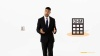••••••••••## Avoid failure: Do not ignore the transmission line effects!

A PCB transmission line is a type of interconnection used for moving signals from their transmitters to their receivers on a printed circuit board. It is composed of two conductors: a signal trace and a return path, which is usually a ground plane. The volume between the two conductors is made up of the PCB dielectric material.

It is crucial not to ignore the transmission line effects in order to avoid signal reflections, crosstalk, electromagnetic noise and other issues which could severely impact the signal quality of your board and cause errors.#### Signal speed and propagation delay

At high frequencies, transmission lines need to have a controlled impedance to predict the behavior of the signals and avoid signal reflections, crosstalk, electromagnetic noise, etc. which could damage the signal quality and cause errors.

This is the reason why you need to know at which speed signals propagate on transmission lines and the time they take to do so. We will give you a few equations to calculate the signal speed and the propagation delay for both striplines and microstrips.#### Estimate the rise/fall time from data transfer rate

The data transfer rate (DTR) is measured in bits per second (bps or bits/sec or b/s) and the clock frequency (Fclock) in Hz. DTR is usually ≥ 2Fclock. Henceforth, it will be safe to use the following rule: DTR = 2Fclock.

If we don’t know the signal rise/fall time, we can use the following rule.

## How to analyze a PCB transmission line

•#### A transmission line segment

A transmission line is a large number of infinitesimal length segments, and each segment can be analyzed by network theory concepts at a particular point in space and time, ignoring the travel time in the infinitesimal segment as its length is extremely small. In this analysis technique, we will be dealing with quantities like voltages and currents, and line parameters like resistance, inductance, capacitance, and conductance.

•#### The characteristic impedance

If we look at an infinite transmission line of characteristic impedance Z0 from the left side at any point, we can see an impedance of Z0. Therefore, if we take a finite length transmission line of impedance Z0 and terminate it on the right by an impedance of value Z0 , and if we look at the finite transmission line from the left, it will appear as an infinite transmission of impedance Z0 from the impedance perspective above.

## We have the design tools you needTake a Peek

## Complete the form below to get your design guide##### "Sierra makes it easy to place orders and offers quality and quick turn for our prototype boards."

Bruce Feige